# Fun with Wilbur, Vol. 6 – Islands

Sometimes you need a quick island and don’t feel much like working at it. It’s simple enough.

## Calculate a Height Field

Filter>>Noise>>Fractal Noise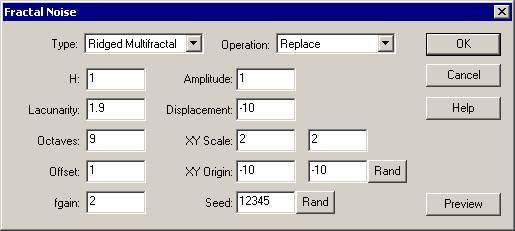This yields the basic height field that has been used for most of these tutorials so far. It’s not terribly interesting.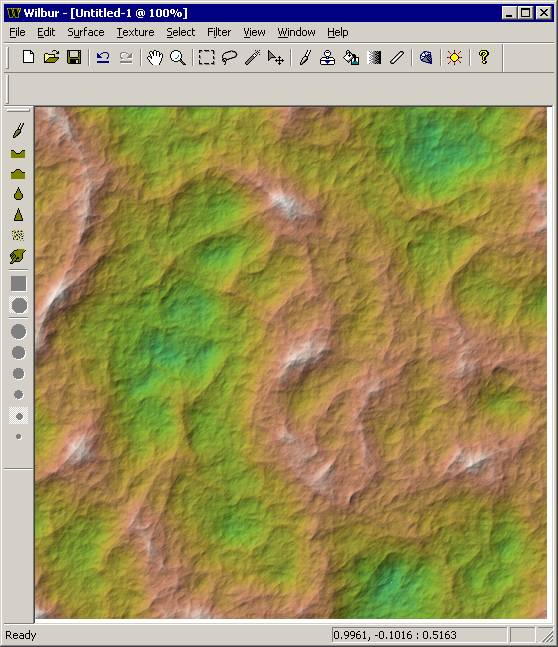## Ensure a known altitude range

Filter>>Mathematical>>Span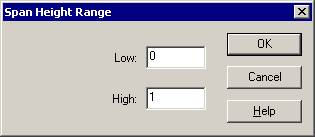The math we’re about to apply works better if the surface is in the range of 0 to 1. It will generate not quite islands if the surface has negative values. Interesting, but not the point of this discussion.

## Force to an Island

Filter>>Calculate Height Field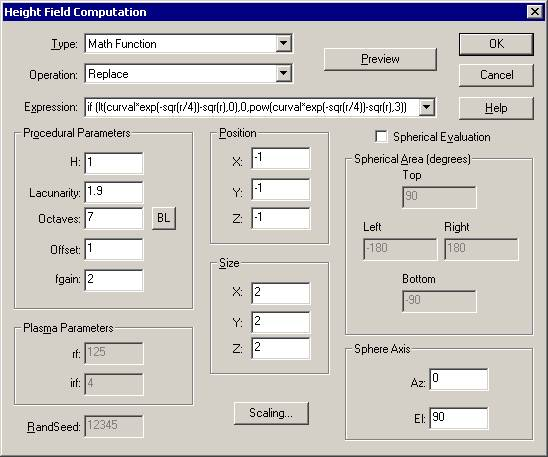Simple, right? The results are acceptable for basic island-type purposes: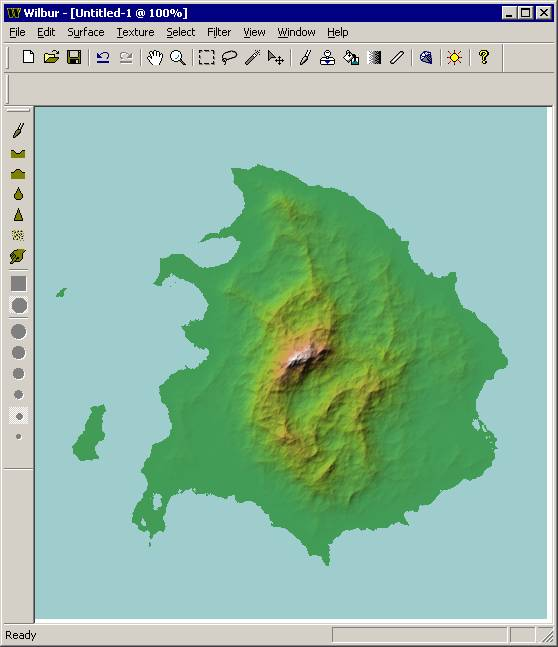Some other examples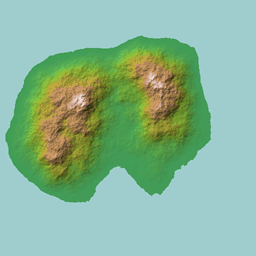Applying the concepts of basin fill and incise flow as described in other Fun with Wilbur tutorials works well here, too: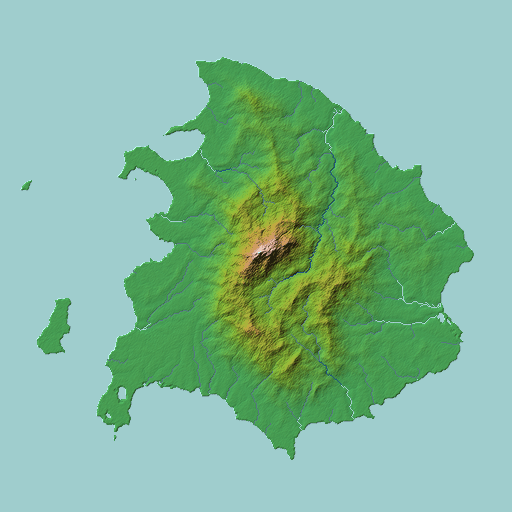## WTF?(Or, how it works)

That step there about “Force to an Island” probably needs a little explaining. The idea is that we take the basic noise field and multiply it by a shape that will determine the overall result. The shape we’re using is a nicely round and symmetric one (shown below):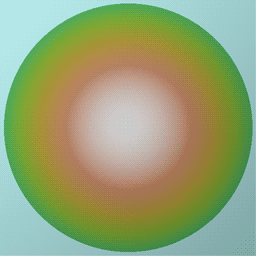Parts in blue will be forced down under the water and the other parts will be shaped to follow the dome.

But that’s not all that’s happening in there. Let’s look closely at the Calculate Height Field dialog:The Type of Math Function indicates that we’ll be doing math rather than just calculating noise. The Operation of Replace indicates a simple replacement. Spherical Position off, Position of -1, -1, -1, and Size of 2, 2, 2 means that all computations will take place in a space going from -1 to +1 in the X, Y, and Z directions. The text listed in Expression is where the magic happens. Nothing else here is important.

### Expression Analysis

Wilbur has a fully-fledged expression parser and lots of built-in functions (see the documentation for a full list). The expression “if (lt(curval*exp(-sqr(r/4))-sqr(r),0),0,pow(curval*exp(-sqr(r/4))-sqr(r),3))” is a bit intimidating, but it’s just several things mashed together. It really reads “if the shaped surface value is less than 0 then use the value of 0 to make the result into ocean, else use the shaped surface value tweaked by an exponential function to make the land area a pointier version of the original value.”

The “if(cond, expr1,expr2)” function uses a conditional expression to select between two other expressions. In this case, that means that we’re using the conditional expression “lt(curval*exp(-sqr(r/4))-sqr(r),0)” to pick between the expressions “0” and “pow(curval*exp(-sqr(r/4))-sqr(r),3)”.

Quick aside - The “lt(a,b)” function means “1 if a is less than b else 0”, the “curval” function returns the altitude of the surface at the current point, the “exp(a)” function yields 2.7182818284590452353602874713527 raised to the power  of a, the “sqr(a)” means “a*a”, the “r” function is shorthand for “square root of (x*x+y*y)”, and “pow(a,b)” means “raise a to the b power”.

The expression “curval*exp(-sqr(r/4))” gives the result of the current surface shaped by a Gaussian envelope (that round dome-thing used to make the island go into the ocean around the edges).

Keeping the middle and flattening the edges isn’t all that’s needed to get a good island – it’s also important to force the edges down. Subtracting the radius value will work nicely, explaining the full “curval*exp(-sqr(r/4))-sqr(r)” expression that gives the basic shaped noise.

Notice that this expression appears in two places: once in the conditional part of the if and once in the else part of the if.  This duplication is necessary because there is no way to store a value in the expression.

The part that reads “pow(shaped noise, 3)” applies a cubic modifier to the shaped noise. This operation applies the idea that islands tend to be flatter near the shore. Changing the 3 to other values will give different results as shown below: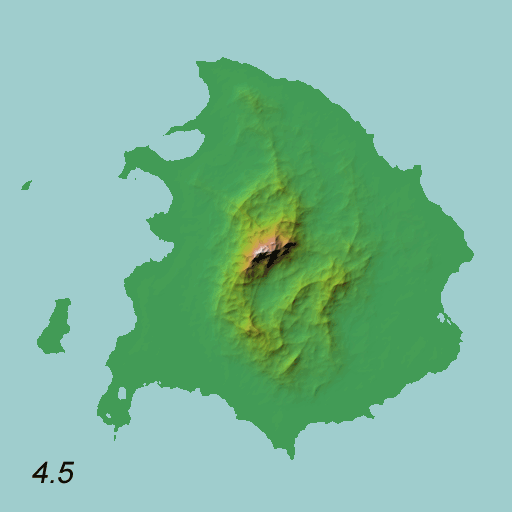I’m not sure all of that was explained as well as it needed to be, but it’s a start.